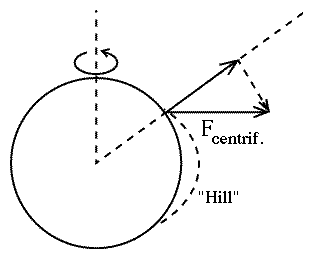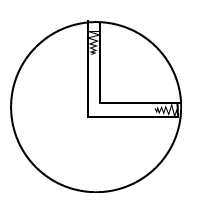# (24a)   The Rotating EarthIndex23a. The Centrifugal Force23b. Loop-the-Loop24a.The Rotating Earth24b. Rotating Frames

The SunS-1. Sunlight & EarthS-1A. WeatherS-1B. Global ClimateS-2.Solar LayersS-3.The Magnetic SunS-3A. Interplanetary
Magnetic FieldsS-4. Colors of Sunlight

### The reduction of effective gravity by the Earth's rotation

One important rotating frame is the surface of the Earth, rotating with a period of about 24 hours--more accurately, 23 hrs 56.07 min or 86164 seconds. If the equatorial radius of Earth is 6378 kilometers, the circumference comes to 40074 kilometers--slightly more than the 40,000 kilometers supposed to be the pole-to-pole circumference, implied by the definition of "one meter." The difference comes because the Earth bulges out at its equator. The velocity of the equator then is

40074 / 86164 = 0.4651 kilometers = 465.1 meter/sec

That velocity is directed eastwards and is significantly faster than the speed of sound, which is about 335 m/s. Space rockets from Cape Canaveral need to attain about 8 km/s (in the frame of reference of the non-rotating center of the Earth ) to achieve orbit, so to give them a favorable starting velocity, such rockets are generally launched eastwards.

To derive the centrifugal acceleration on the equator (i.e. the force in Newtons on one gram mass, rotating with the Earth), we calculate in meters and seconds

v2 / r = (465.1)2 / 6378000 = 216318 / 6378000 = 0,03392 m/s2

Comparing this to the acceleration of gravity--say 9.81 m/s2--it is only 0.00346 or 0.346%. Effective gravity on the equator is reduced by the rotation, but only by about 1/3 of a percent

### The bulge of the Earth's equatorAssuming the Earth is exactly spherical, we expect gravity to always point towards the center of Earth. However, the centrifugal force is perpendicular to the axis of the Earth. Except on the equator, therefore, it is not exactly opposed to gravity, but adds a small horizontal vector component, pointing towards the equator (dashed arrow in the figure). As a result, not only is effective gravity weakened, but its direction is modified--instead of pointing to the center of the Earth, is slants (ever so slightly) towards the equator.

Does this mean that if you placed a perfect ball on a very smooth horizontal surface, gravity would make it roll equatorward...? Suppose it was so. That same force would also act on the water of the ocean and make it flow equatorwards, and even the solid Earth might deform!

How long would this go on? Well, until the equatorial pile-up of material forms a "hill" around the Earth, rising slightly towards the equator, where its top would be. No more flow towards the equator would occur once the slope of the ground, as modified by the hill, would be exactly perpendicular to the effective direction of (modified) gravity. With such a slope, a perfect ball placed on a perfectly horizontal surface would no longer try to roll anywhere, and forces on oceans and on land would no longer try to move matter horizontally.

By now you should realize what this gives us--an explanation to the equatorial bulge of the Earth! That bulge is nothing but the "hill" described above, and the shape of the surface is such that the ground is always perpendicular to the effective gravity

Jupiter rotates in just under 10 hours and has an equatorial radius about 11 times that of Earth, creating a much stronger centrifugal force. As a result, in spite of a surface gravity 2.64 times stronger than Earth's, Jupiter is much more oblate, by about 6.5%.The oblateness of Jupiter was noted through the telescope by Giovanni Cassini (1625-1712), director of the Paris observatory, However, he and his son also claimed that Earth was different, that it bulged at the poles (more like a lemon than like a grapefruit). They based their claim on measurements on the ground, which suggested lines of latitude became closer together as one approached the pole.

Their result was later disproved by more accurate observations, but even before that, Newton by the following simple argument showed this could not be so. Assume Earth is a sphere, and imagine two deep holes extending to its center (see drawing), meeting there and filled with water. If Earth did not rotate, one may have expected the height of each water column to be the same: at the center of the Earth, by symmetry, each column would push down with the same weight, creating an equilibrium.

On a rotating Earth, however, the centrifugal force acts to reduce the weight of the water in the equatorial hole, and the water would rise there to greater height. Newton then argued that water anywhere would rise to the same level

 ...if our earth were not a little higher around the equator than at the poles, the seas would subside at the poles and, by ascending in the region of the equator, would flood everything there.

For more about the bulge of the Earth (including a more advanced mathematical treatment) see web site "The Bulging Earth".

### Jet planes above the equator

(Thanks to John Lowry for this neat example!)

The centrifugal force is a useful concept when figuring out equilibria in a rotating frame of reference, cases where forces balance exactly and no motion occurs.

To calculate motion in a rotating frame is much more complicated, and in general is beyond the level of this presentation. All that will be done in this section and the one that follows is point out some complications.     Let us assume the reduction of effective gravity is the same at jet-plane altitude--a small difference exists, but does not affect the argument below. Two jet airplanes fly along the equator at equal speeds--one eastwards, the other westwards. Since they fly in circles around the center of Earth, the effective gravity aboard each of them is further changed. Do both sense the same change?

You might think so, but it isn't true.

It would be the same if the Earth did not rotate (neglect here the attraction of the Sun and the rotation of the Earth around it, two effects which about balance). Without Earth rotation, in the frame of the universe, the eastward and westward motions are completely symmetric.

Actually, however, the speeds of the jets are measured relative to that atmosphere, which itself also rotates around the center of the Earth. Viewed from the frame of the universe, the speed of the Eastward jet is added to the rotation of the atmosphere, the one of the westward jet is subtracted.

Suppose these are two supersonic Concorde airliners, flying at 465.1 meter/sec. Rather than study the motion in a rotating frame, which may or may not give correct answers, we can always go back to the non-rotating frame of the universe. In that frame, the rotational velocity of the westward jet is zero, and it senses no effective reduction of gravity. In the same frame, the eastward jet has twice the velocity, and since the centrifugal force is proportional to the velocity squared (v2), it senses four times the centrifugal force. Effective gravity aboard that jet is reduced by four times the amount felt on the ground, or 1.384%.

### What it means

The above example suggests that we better watch out before applying Newton's laws in a rotating frame.

Newton's first law certainly does not hold--a body not acted on by any force will not stay at rest but will fly off at a tangent. One can salvage this part of the law by adding a centrifugal force in any calculation of balanced forces. But if such forces are not balanced, if they cause motion in the rotating frame, then a modified form of Newton's laws must be used. They do exist, but are beyond the level of this course. The above example suggests some of the problems of rotating frames, and more examples are in the next section, which tells about the Coriolis effect.

You and I, and anyone else on Earth, live in a rotating frame of reference. Should we therefore refrain from using Newton's equations in everyday life? It turns out that for motions on a scale much smaller than the size of Earth, at moderate velocities, the effects are negligible.

One example concerns the famous question of whether draining water in kitchen sinks on opposite sides of the equator swirls in opposite directions, as is sometimes claimed. It turns out (see next section) that the effect in principle exists, but is much too small to affect observations. In the world wars, gunners firing big cannons corrected their aim (slightly) to account for the Earth's rotation, but you and I can usually ignore it, except for the modification of gravity by the centrifugal force.

Questions from Users:   What would happen if Earth rotated faster?
And in the opposite direction:  What if the rotation of Earth could be stopped?.
Also: ***  Acceleration due to gravity.
***  Does the Earth's core share the Earth's rotation?.
***  If the Earth's Rotation would Stop...
***  If the Earth's Rotation would Change...
***  What makes the Earth rotate?

Author and Curator:   Dr. David P. Stern
Mail to Dr.Stern:   stargaze("at" symbol)phy6.org .

Last updated: 9-22-2004
Reformatted 25 March 2006